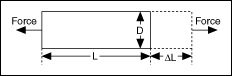# Measuring Strain

NI-DAQmx Help

Edition Date: January 2019

Part Number: 370466AH-01

»View Product InfoDownload Help (Windows Only)

Strain (ε) is the amount of deformation of a body due to an applied force. Specifically, strain is the fractional change in length, as shown in the following figure.Strain can be positive (tensile) or negative (compressive). Although dimensionless, strain is sometimes expressed in units such as in./in. or mm/mm. In practice, the magnitude of measured strain is very small. Therefore, strain is often expressed as microstrain (µε).

When a uniaxial force strains a bar, as in the preceding figure, a phenomenon known as Poisson Strain causes the girth of the bar, D, to contract in the transverse direction, which is perpendicular to the force. The magnitude of this transverse contraction is a material property indicated by its Poisson's Ratio. The Poisson's Ratio of a material is the negative ratio of the strain in the transverse direction to the strain in the axial direction, which is parallel to the force. Poisson's Ratio for steel, for example, ranges from 0.25 to 0.3.

To measure strain, you can use one or more strain gages in a Wheatstone bridge in one of several bridge configurations. Refer to Signal Conditioning Requirements for Bridge-Based Sensors for more information about strain gages and bridge configurations.

Making Signal Connections

Creating a Program

Examples